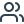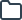Statistics at Ariel University Center Of Samaria | Flashcards & Summaries

Suggested languages for you:# Lernmaterialien für Statistics an der Ariel University Center of Samaria

Greife auf kostenlose Karteikarten, Zusammenfassungen, Übungsaufgaben und Altklausuren für deinen Statistics Kurs an der Ariel University Center of Samaria zu.

TESTE DEIN WISSEN

Define expected value

Lösung anzeigen
TESTE DEIN WISSEN

The expected value of a random variable is the sum of all the values of the random variable multiplied by their probabilities.

Jenny = 1, Lora = 2, Sanya = 3

1 = 0.1

2 =  0.5

3 = 0.3

expected_one_to_call = 1*0.1 + 2*0.5 + 3*0.3 = 2

Our expected value is 2, (Lora)

Lösung ausblenden
TESTE DEIN WISSEN

The solution for graphical represantation of discrete variables is histogram. But that doesn't work for the continues variables. What are the solutions?

Lösung anzeigen
TESTE DEIN WISSEN

One way to visualize the distribution of continuous variables is to divide the set of possible values into intervals and count the number of values in each interval.

When plotting a histogram in pandas, you can set the number of intervals (bins) and set their boundaries explicitly:

data.hist( bins = [value1, value2, value.... valueN])

To overcome the difficulties of creating a histogram for a continuous variable, we can use a slightly different technique that represents frequency not as the height of a column, but as its area (the length of the interval times the height of the column). This area is the frequency of the continuous variable, and the height of the column is the frequency density. A histogram that uses frequency density is called a density histogram.  To estimate how many values fall in a particular interval, take two values and find the total area of the density histograms between them. The number you get will be an estimate of the number of values in that interval.

Lösung ausblenden
TESTE DEIN WISSEN

Data with negative skew (skewed to the left) has ...

Lösung anzeigen
TESTE DEIN WISSEN

Data with negative skew (skewed to the left) has a mean that is less than the median. The data will have more values below the mean than above it.

Lösung ausblenden
TESTE DEIN WISSEN

How do you call the std func in python. What library do you use?

Lösung anzeigen
TESTE DEIN WISSEN

standard_deviation = np.std(dataset)

Lösung ausblenden
TESTE DEIN WISSEN

Data with positive skew (skewed to the right) has...

Lösung anzeigen
TESTE DEIN WISSEN

Data with positive skew (skewed to the right) has a mean that is greater than the median. The data will have more values greater than the mean than below it.

Lösung ausblenden
TESTE DEIN WISSEN

Find dispersion of data

Lösung anzeigen
TESTE DEIN WISSEN

import numpy as np

np.var(data)

Lösung ausblenden
TESTE DEIN WISSEN

What is Frequency density ?

Lösung anzeigen
TESTE DEIN WISSEN

Frequency density — a value equal to the height of a histogram column whose area reflects the relative frequency of a continuous variable.

Lösung ausblenden
TESTE DEIN WISSEN

Define a discrete variable.

Lösung anzeigen
TESTE DEIN WISSEN

A  discrete variable is any variable that is not continuous on any range (for example a variable that takes the integer values from 0 to 100).

Lösung ausblenden
TESTE DEIN WISSEN

What is a Density histogram

Lösung anzeigen
TESTE DEIN WISSEN

Density histogram — a histogram that uses frequency density.

Lösung ausblenden
TESTE DEIN WISSEN

What is μ?

Lösung anzeigen
TESTE DEIN WISSEN

The mean, often denoted by the Greek letter mu, μ.

Lösung ausblenden
TESTE DEIN WISSEN

Build histograms of data with set interval boundaries 16,18,20,58,100

Lösung anzeigen
TESTE DEIN WISSEN

data.hist(bins=[16,18,20,58,100 ])

Lösung ausblenden
TESTE DEIN WISSEN

If mean > median

is it a positive or negative skew?

Lösung anzeigen
TESTE DEIN WISSEN

if the mean is greater than the median, then the data has positive skew. Similarly, if the mean is less than the median, then the data has negative skew.

Lösung ausblenden•354 Karteikarten
•38 Studierende
•0 Lernmaterialien

## Beispielhafte Karteikarten für deinen Statistics Kurs an der Ariel University Center of Samaria - von Kommilitonen auf StudySmarter erstellt!

Q:

Define expected value

A:

The expected value of a random variable is the sum of all the values of the random variable multiplied by their probabilities.

Jenny = 1, Lora = 2, Sanya = 3

1 = 0.1

2 =  0.5

3 = 0.3

expected_one_to_call = 1*0.1 + 2*0.5 + 3*0.3 = 2

Our expected value is 2, (Lora)

Q:

The solution for graphical represantation of discrete variables is histogram. But that doesn't work for the continues variables. What are the solutions?

A:

One way to visualize the distribution of continuous variables is to divide the set of possible values into intervals and count the number of values in each interval.

When plotting a histogram in pandas, you can set the number of intervals (bins) and set their boundaries explicitly:

data.hist( bins = [value1, value2, value.... valueN])

To overcome the difficulties of creating a histogram for a continuous variable, we can use a slightly different technique that represents frequency not as the height of a column, but as its area (the length of the interval times the height of the column). This area is the frequency of the continuous variable, and the height of the column is the frequency density. A histogram that uses frequency density is called a density histogram.  To estimate how many values fall in a particular interval, take two values and find the total area of the density histograms between them. The number you get will be an estimate of the number of values in that interval.

Q:

Data with negative skew (skewed to the left) has ...

A:

Data with negative skew (skewed to the left) has a mean that is less than the median. The data will have more values below the mean than above it.

Q:

How do you call the std func in python. What library do you use?

A:

standard_deviation = np.std(dataset)

Q:

Data with positive skew (skewed to the right) has...

A:

Data with positive skew (skewed to the right) has a mean that is greater than the median. The data will have more values greater than the mean than below it.

Q:

Find dispersion of data

A:

import numpy as np

np.var(data)

Q:

What is Frequency density ?

A:

Frequency density — a value equal to the height of a histogram column whose area reflects the relative frequency of a continuous variable.

Q:

Define a discrete variable.

A:

A  discrete variable is any variable that is not continuous on any range (for example a variable that takes the integer values from 0 to 100).

Q:

What is a Density histogram

A:

Density histogram — a histogram that uses frequency density.

Q:

What is μ?

A:

The mean, often denoted by the Greek letter mu, μ.

Q:

Build histograms of data with set interval boundaries 16,18,20,58,100

A:

data.hist(bins=[16,18,20,58,100 ])

Q:

If mean > median

is it a positive or negative skew?

A:

if the mean is greater than the median, then the data has positive skew. Similarly, if the mean is less than the median, then the data has negative skew.### Erstelle und finde Lernmaterialien auf StudySmarter.

Greife kostenlos auf tausende geteilte Karteikarten, Zusammenfassungen, Altklausuren und mehr zu.

## Das sind die beliebtesten Statistics Kurse im gesamten StudySmarter Universum

##### Statistics 3

Griffith University

Taif University

##### Statistics 3

Vrije Universiteit Amsterdam

Umea University

##### MCQ Statistics

University of Delhi

## Die all-in-one Lernapp für Studierende

##### Greife auf Millionen geteilter Lernmaterialien der StudySmarter Community zu##### Erstelle Karteikarten und Zusammenfassungen mit den StudySmarter Tools